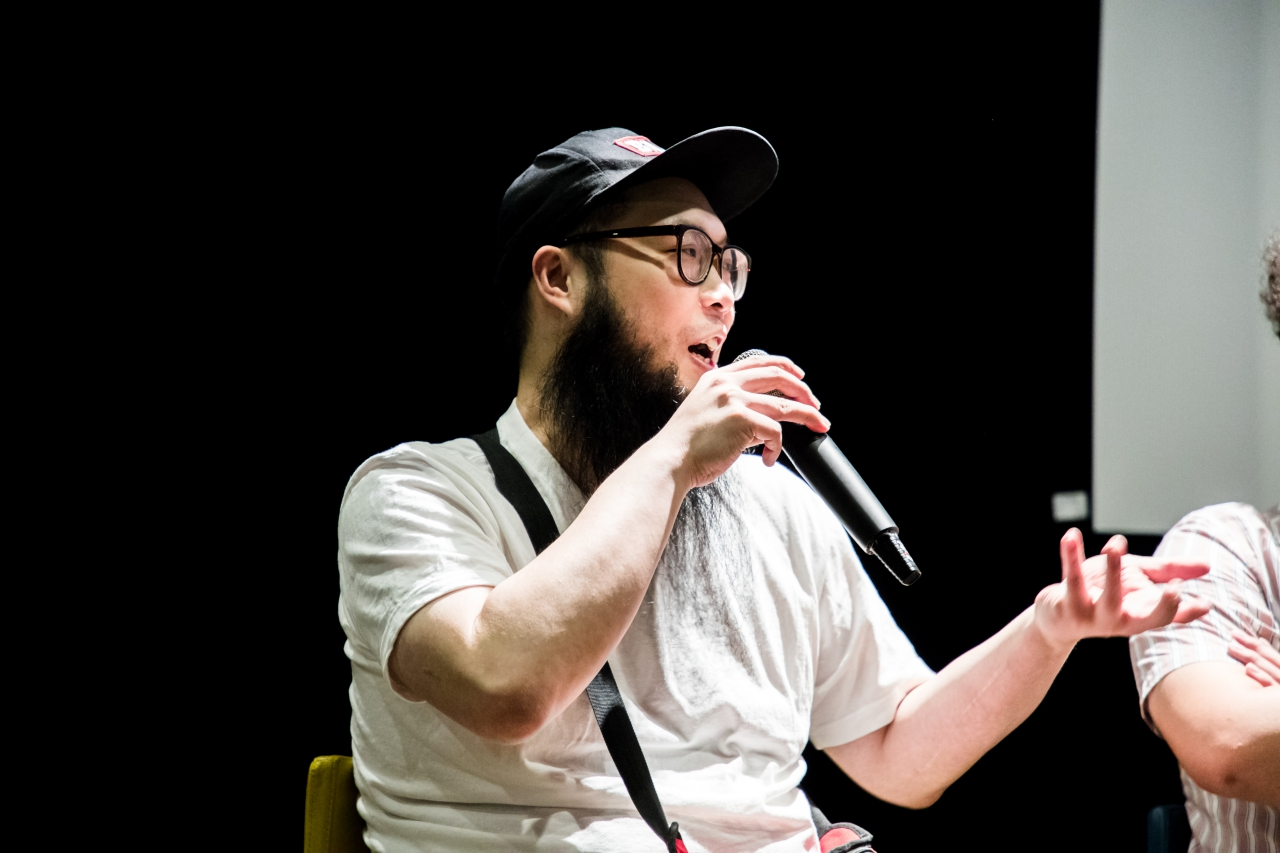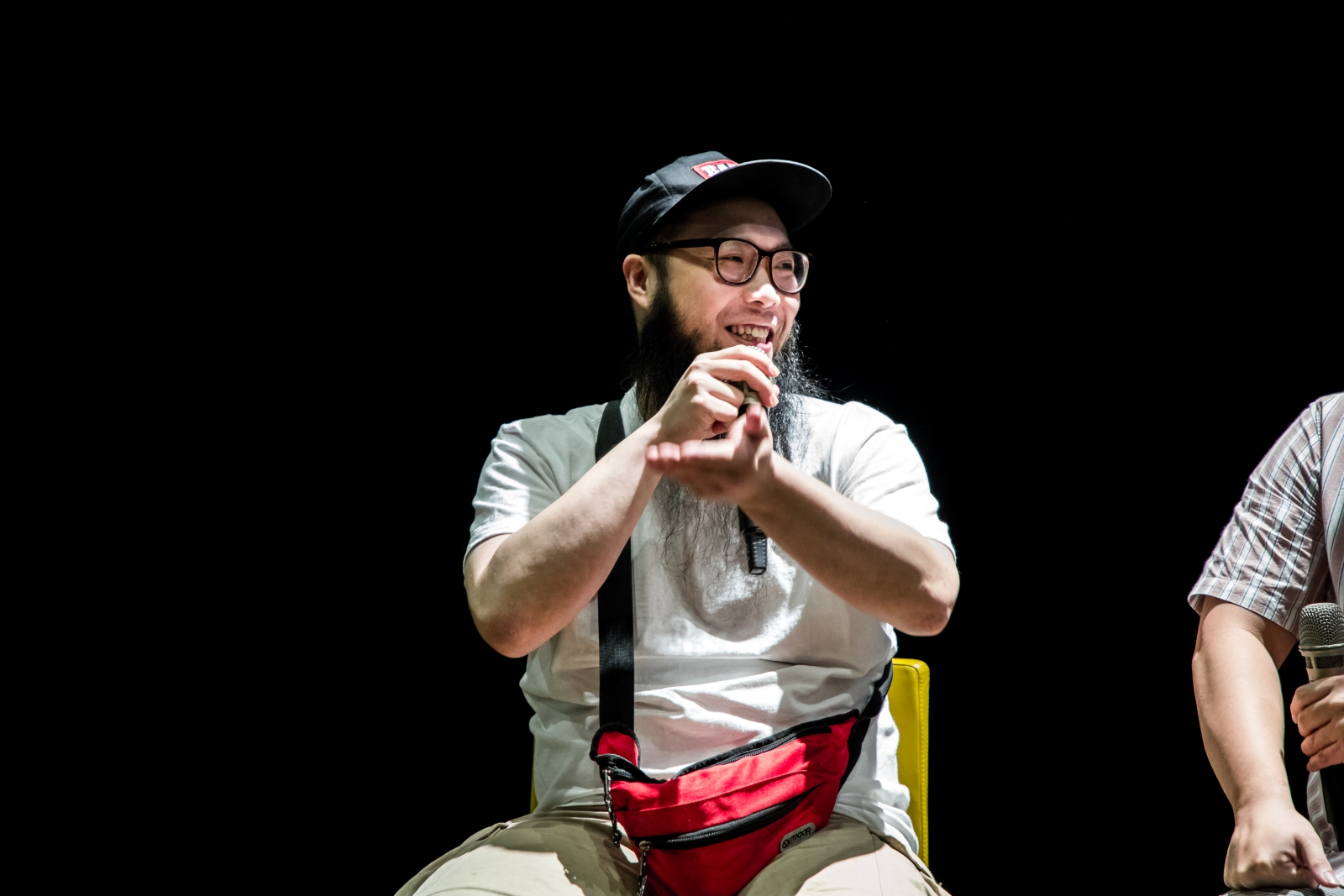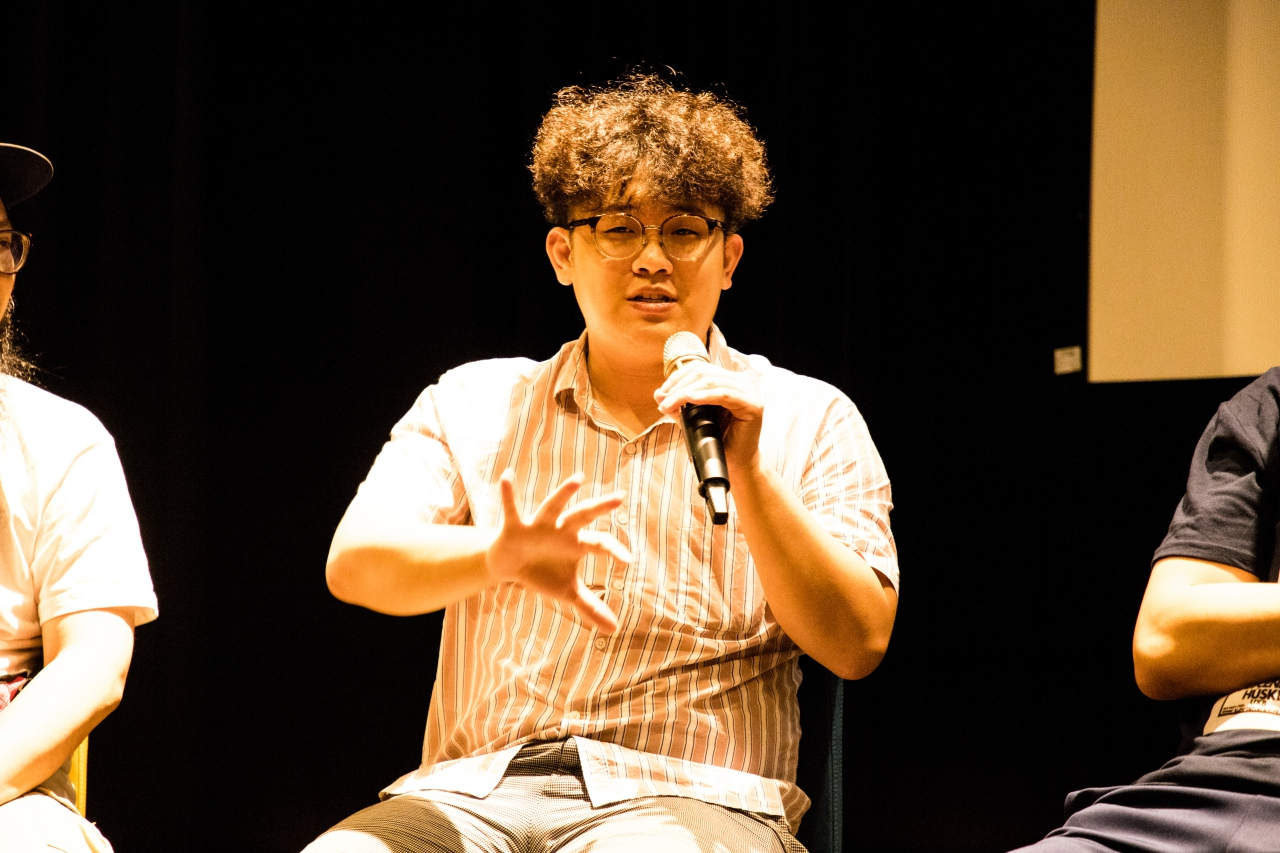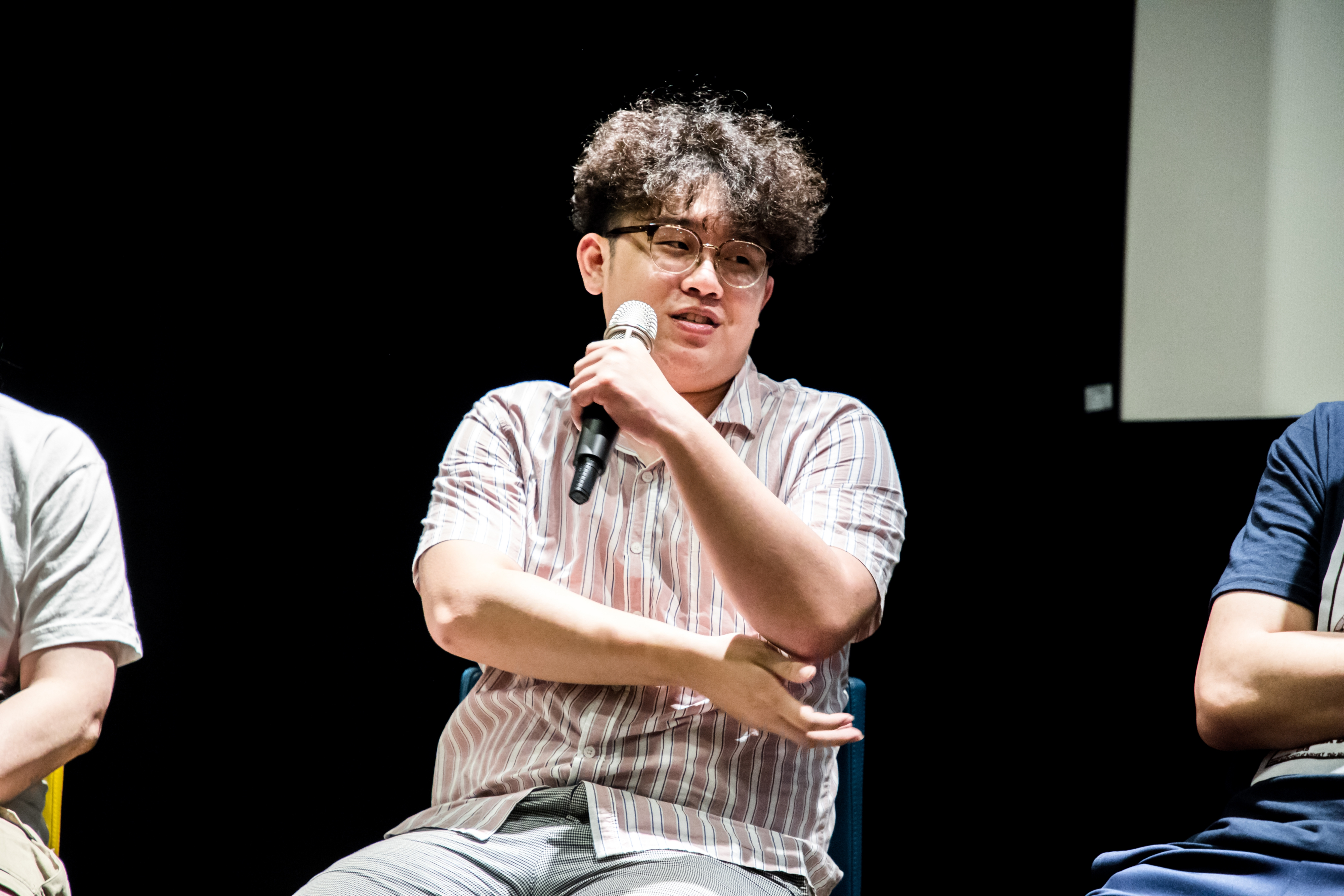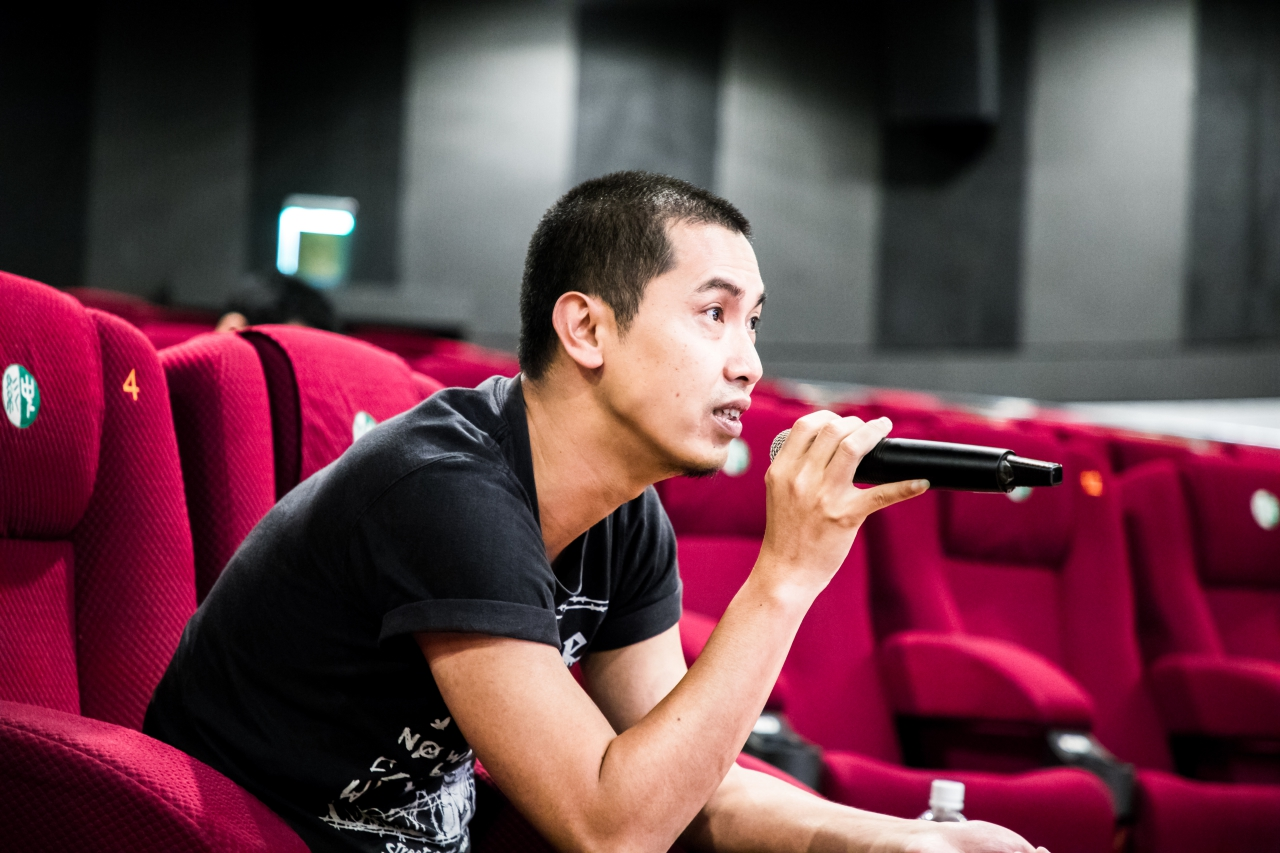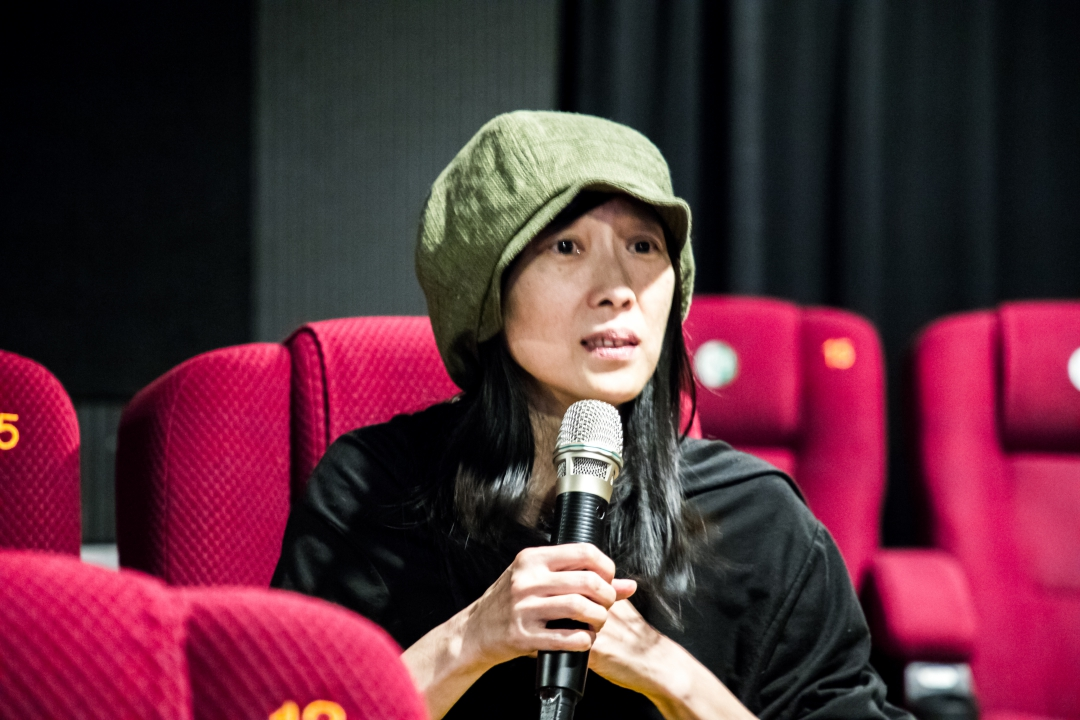（一）導談與介紹

Q:

A:

Q:

A:

Q:

A:

Q:

A:

（二）音樂祭

Q:

A:

Q:

A:

Q:

A:

Q:

A:

Q:

A:

Q:

A:

Q:

A:

Q:

A:

Q:

A:

（三）兩地交流

Q:

A:

Q:

A:

Q:

A:

Q:

A:

（四）結論

（五）觀眾提問

Q:

A:

Q:

A:

Q:

A:

Q:

A:

Q:

A: